1. /
2. CBSE
3. /
4. Class 07
5. /
6. Mathematics
7. /
8. NCERT Solutions for Class...

# NCERT Solutions for Class 7 Maths Exercise 5.2### myCBSEguide App

Download the app to get CBSE Sample Papers 2023-24, NCERT Solutions (Revised), Most Important Questions, Previous Year Question Bank, Mock Tests, and Detailed Notes.

NCERT solutions for Maths Lines and Angles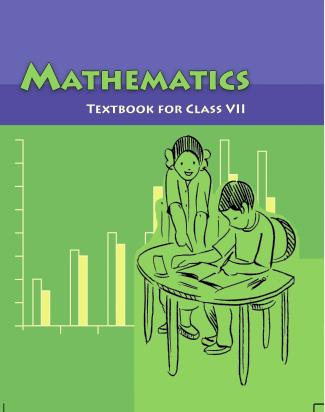## NCERT Solutions for Class 7 Maths Lines and Angles

###### Question 1.State the property that is used in each of the following statements: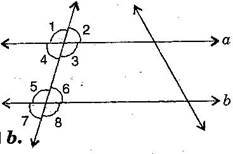1. If {tex}a\parallel b,{/tex} then {tex}\angle {/tex}1 = {tex}\angle {/tex}5.
2. If {tex}\angle {/tex}4 = {tex}\angle {/tex}6, then {tex}a\parallel b.{/tex}
3. If {tex}\angle {/tex}4 + {tex}\angle {/tex}5 + {tex}180^\circ ,{/tex} then {tex}a\parallel b.{/tex}

(i) Given, {tex}a\parallel b{/tex} then {tex}\angle {/tex}1 = {tex}\angle {/tex}5 [Corresponding angles]

If two parallel lines are cut by a transversal, each pair of corresponding angles are equal in measure.

(ii) Given, {tex}\angle {/tex}4 = {tex}\angle {/tex}6, then {tex}a\parallel b{/tex} [Alternate interior angles]

When a transversal cuts two lines such that pairs of alternate interior angles are equal, the lines have to be parallel.

(iii) Given, {tex}\angle {/tex}4 + {tex}\angle {/tex}5 = {tex}180^\circ ,{/tex} then {tex}a\parallel b{/tex} [

When a transversal cuts two lines, such that pairs of interior angles on the same side of transversal are supplementary, the lines have to be parallel.

NCERT Solutions for Class 7 Maths Exercise 5.2

###### Question 2.In the adjoining figure, identify: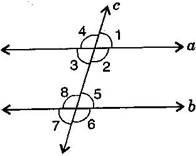1. the pairs of corresponding angles.
2. the pairs of alternate interior angles.
3. the pairs of interior angles on the same side of the transversal.
4. the vertically opposite angles.

(i) The pairs of corresponding angles:

{tex}\angle {/tex}1, {tex}\angle {/tex}5; {tex}\angle {/tex}2, {tex}\angle {/tex}6; {tex}\angle {/tex}4, {tex}\angle {/tex}8 and {tex}\angle {/tex}3, {tex}\angle {/tex}7

(ii) The pairs of alternate interior angles are:

{tex}\angle {/tex}3, {tex}\angle {/tex}5 and {tex}\angle {/tex}2, {tex}\angle {/tex}8

(iii) The pair of interior angles on the same side of the transversal:

{tex}\angle {/tex}3, {tex}\angle {/tex}8 and {tex}\angle {/tex}2, {tex}\angle {/tex}5

(iv) The vertically opposite angles are:

{tex}\angle {/tex}1, {tex}\angle {/tex}3; {tex}\angle {/tex}2, {tex}\angle {/tex}4; {tex}\angle {/tex}6, {tex}\angle {/tex}8 and {tex}\angle {/tex}5, {tex}\angle {/tex}7

NCERT Solutions for Class 7 Maths Exercise 5.2

###### Question 3.In the adjoining figure, {tex}p\parallel q.{/tex} Find the unknown angles.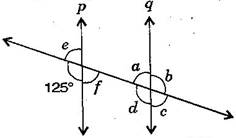Given, {tex}p\parallel q{/tex} and cut by a transversal line.

{tex}\because {/tex} {tex}125^\circ + e = 180^\circ {/tex} [Linear pair]

{tex}\therefore {/tex} {tex}e = 180^\circ – 125^\circ = 55^\circ {/tex} ……….(i)

Now {tex}e = f = 55^\circ {/tex} [Vertically opposite angles]

Also {tex}a = f = 55^\circ {/tex} [Alternate interior angles]

{tex}a + b = 180^\circ {/tex} [Linear pair]

{tex} \Rightarrow {/tex} {tex}55^\circ + b = 180^\circ {/tex} [From eq. (i)]

{tex} \Rightarrow {/tex} {tex}b = 180^\circ – 55^\circ = 125^\circ {/tex}

Now {tex}a = c = 55^\circ {/tex} and {tex}b = d = 125^\circ {/tex} [Vertically opposite angles]

Thus, {tex}a = 55^\circ ,b = 125^\circ ,c = 55^\circ ,d = 125^\circ ,e = 55^\circ {/tex} and {tex}f = 55^\circ .{/tex}

NCERT Solutions for Class 7 Maths Exercise 5.2

###### Question 4.Find the values of {tex}x{/tex} in each of the following figures if {tex}l\parallel m.{/tex}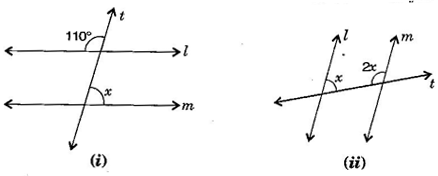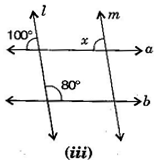(i) Given, {tex}l\parallel m{/tex} and {tex}t{/tex} is transversal line.

{tex}\therefore {/tex} Interior vertically opposite angle between lines {tex}l{/tex} and {tex}t = 110^\circ .{/tex}

{tex}\therefore {/tex} {tex}110^\circ + x = 180^\circ {/tex} [Supplementary angles]

{tex} \Rightarrow {/tex} {tex}x = 180^\circ – 110^\circ = 70^\circ {/tex}

(ii) Given, {tex}l\parallel m{/tex} and {tex}t{/tex} is transversal line.

{tex}x + 2x = 180{/tex} [Interior opposite angles]

{tex} \Rightarrow {/tex} {tex}3x = 180^\circ {/tex} {tex} \Rightarrow {/tex} {tex}x = \frac{{180^\circ }}{3} = 60^\circ {/tex}

(iii) Given, {tex}l\parallel m{/tex} and {tex}a\parallel b{/tex}.

{tex}x = 100^\circ {/tex} [Corresponding angles]

NCERT Solutions for Class 7 Maths Exercise 5.2

###### Question 5.In the given figure, the arms of two angles are parallel. If {tex}\Delta {\text{ABC = 70}}^\circ {\text{,}}{/tex} then find: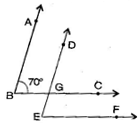(i) DGC

(ii) DEF

(i) Given, AB {tex}\parallel {/tex} DE and BC is a transversal line and {tex}\angle {\text{ABC}} = 70^\circ {/tex}

{tex}\because {/tex} {tex}\angle {/tex}ABC = {tex}\angle {/tex}DGC [Corresponding angles]

{tex}\therefore {/tex} {tex}\angle {/tex}DGC = {tex}70^\circ {/tex} ……….(i)

(ii) Given, BC {tex}\parallel {/tex} EF and DE is a transversal line and {tex}\angle {\text{DGC}} = 70^\circ {/tex}

{tex}\because {/tex} {tex}\angle {/tex}DGC = {tex}\angle {/tex}DEF [Corresponding angles]

{tex}\therefore {/tex} {tex}\angle {/tex}DEF = {tex}70^\circ {/tex} [From eq. (i)]

NCERT Solutions for Class 7 Maths Exercise 5.2

###### Question 6.In the given figures below, decide whether {tex}l{/tex} is parallel to {tex}m.{/tex}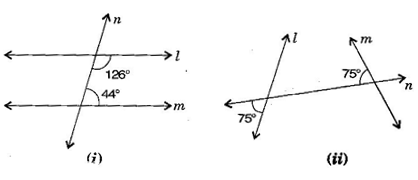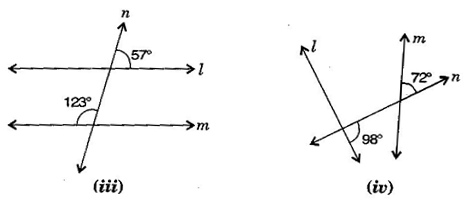(i) {tex}126^\circ + 44^\circ = 170^\circ {/tex}

{tex}l{/tex} is not parallel to {tex}m{/tex} because sum of interior opposite angles should be {tex}180^\circ .{/tex}

(ii) {tex}75^\circ + 75^\circ = 150^\circ {/tex}

{tex}l{/tex} is not parallel to {tex}m{/tex} because sum of angles does not obey the property of parallel lines.

(iii) {tex}57^\circ + 123^\circ = 180^\circ {/tex}

{tex}l{/tex} is parallel to {tex}m{/tex} due to supplementary angles property of parallel lines.

(iv) {tex}98^\circ + 72^\circ = 170^\circ {/tex}

{tex}l{/tex} is not parallel to {tex}m{/tex} because sum of angles does not obey the property of parallel lines.

## NCERT Solutions for Class 7 Maths Exercise 5.2

NCERT Solutions Class 7 Maths PDF (Download) Free from myCBSEguide app and myCBSEguide website. Ncert solution class 7 Maths includes text book solutions from Class 7 Maths Book . NCERT Solutions for CBSE Class 7 Maths have total 15 chapters. 7 Maths NCERT Solutions in PDF for free Download on our website. Ncert Maths class 7 solutions PDF and Maths ncert class 7 PDF solutions with latest modifications and as per the latest CBSE syllabus are only available in myCBSEguide.

## CBSE app for Students

To download NCERT Solutions for Class 7 Maths, Social Science Computer Science, Home Science, Hindi English, Maths Science do check myCBSEguide app or website. myCBSEguide provides sample papers with solution, test papers for chapter-wise practice, NCERT solutions, NCERT Exemplar solutions, quick revision notes for ready reference, CBSE guess papers and CBSE important question papers. Sample Paper all are made available through the best app for CBSE students and myCBSEguide website.Test Generator

Create question paper PDF and online tests with your own name & logo in minutes.myCBSEguide

Question Bank, Mock Tests, Exam Papers, NCERT Solutions, Sample Papers, Notes

### 1 thought on “NCERT Solutions for Class 7 Maths Exercise 5.2”

1. Extra questions so boring……